# Factor Trinomial: Grid Method

Using a grid can be a helpful way to factor a trinomial. The objective of factoring a trinomial is to begin with an expression such aswhereandhave no common factors*; and write an equivalent expressionFor exampleThe grid method can also be used with an unreduced quadratic. See ‘method 2’ below.

# Highest Common Factor

In order to use the grid method, we need to be able to take the highest common factor of two terms.

For example, the highest common factor ofandis.

The highest common factor ofandis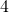.

We can write this using shorthand notation:

HCFHCFReview highest common factor here.

# The Grid Method

In general, the grid is filled out as follows: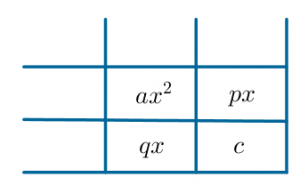Let’s examineWe begin by drawing a 2 by 2 grid, with space on top and on the left as follows: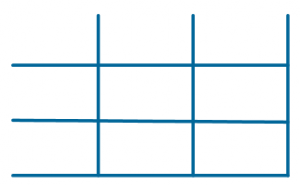We first enter theterm and theterm as follows.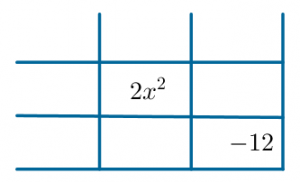We then need to find two numbersandsuch thatIn our example, that isStudy the factor pairs of 24, remembering that to multiply to a negative, one of the factors must be negative. The list includes:. The factor pairworks using:We complete the grid using these two values as follows (either way around):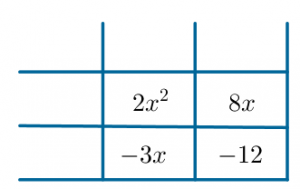If the quadratic is reduced (that is, HCF), we can now simply take the highest common factor from each row/column as follows:

First row: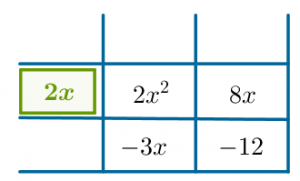Second row: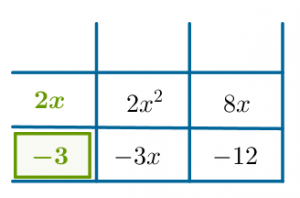First column: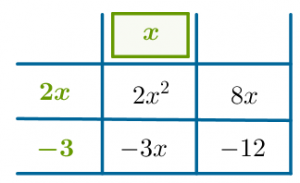Second column: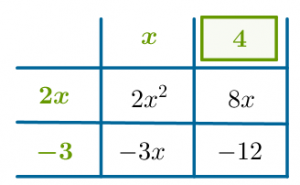Check that each term in the grid is the product of green terms in the same row and column: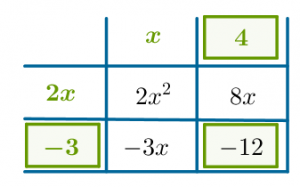On the left, we see our factor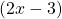and on the top row we see the factor. Therefore,Check by expanding the brackets.

## Method 2

If the quadratic is not reduced, we take the highest common factor from any one row or column, and then complete the remaining boxes as follows:

Highest common factor from any one row or column:What multipliesto get? Complete first column:What multipliesto get? Complete second row: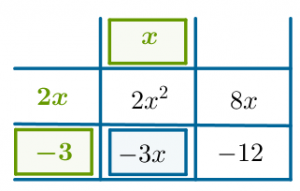What multipliesto get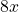? Complete second column.Check the last box works too:If the quadratic was not already reduced, you will see a common factor in at least one of your two factors.

applet link

Why it works